# 基于MaxCompute InformationSchema进行冷门表热门表访问分析

select
inst_id ,
input_tables,
output_tables,
start_time,
end_time
where ds='20190902'limit 100;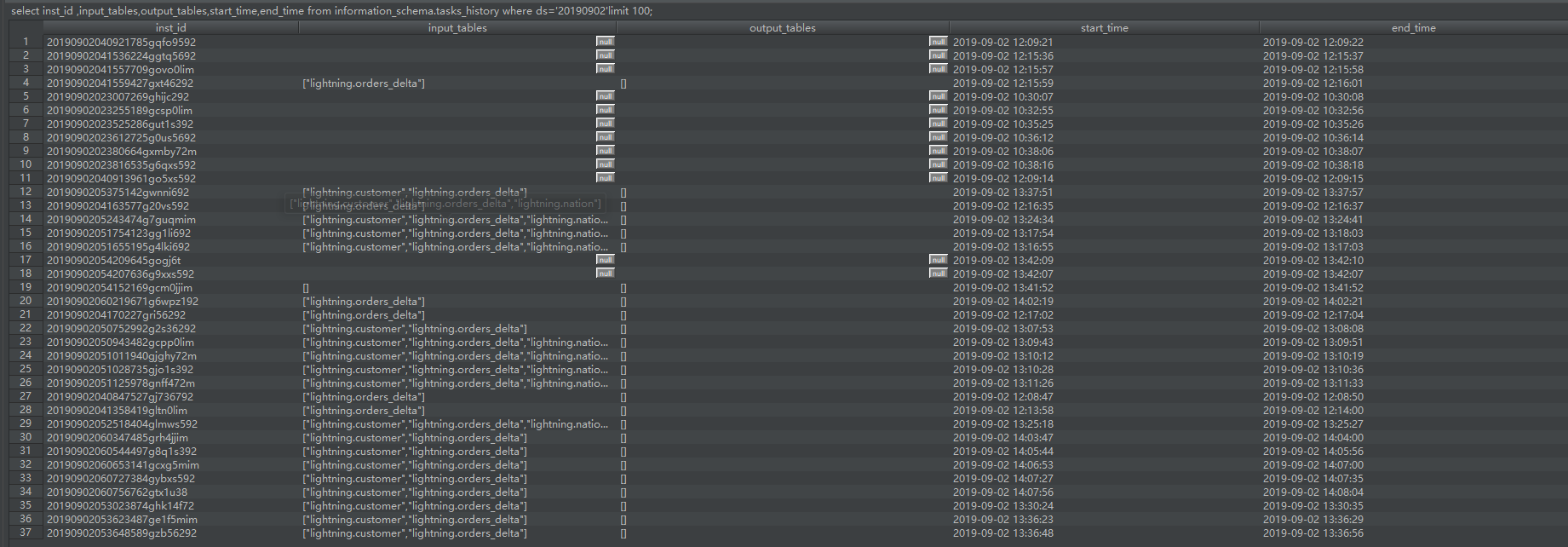["lightning.customer","lightning.orders_delta"]

select
--去掉input_tables 字段中开始和结尾的[]
trans_array(1,",",inst_id,replace(replace(input_tables,"[",""),"]","")) as (inst_id,input_table)
--日期可以根据实际需求去限定，这里以20190902为例
where ds='20190902' limit 100;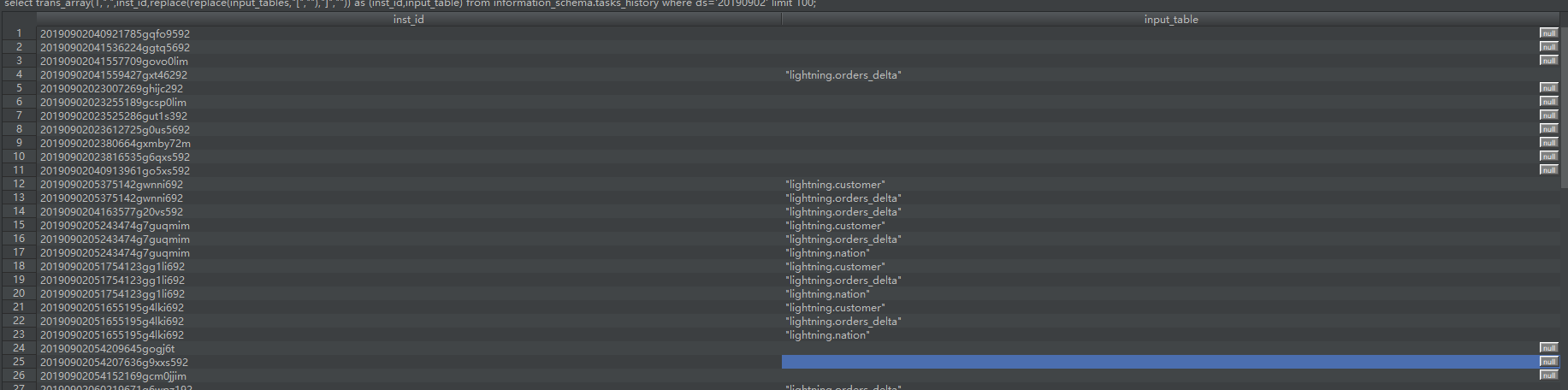2、统计热门表数据SQL编写:
select
--按表名进行统计计算
input_table
from
(
select
--去掉input_tables 字段中开始和结尾的[]
trans_array(1,",",inst_id,replace(replace(input_tables,"[",""),"]","")) as (inst_id,input_table)
where ds='20190902'
) t
group by input_table
limit 1000;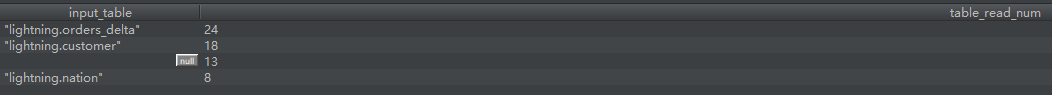3、统计冷门表数据SQL编写:

select
t1.table_schema,
t1.table_name,
--两表关联
FROM information_schema.tables t1
left join(
select
--去掉表名前后的”符号
regexp_replace(t.input_table,""","") as input_table
from
(
select
--去掉input_tables 字段中开始和结尾的[]
trans_array(1,",",inst_id,replace(replace(input_tables,"[",""),"]","")) as (inst_id,input_table)
where ds='20190902' )t
group by input_table
)t2
--关联条件匹配
on concat('your_project_name.',t1.table_name)=t2.input_table
limit 1000;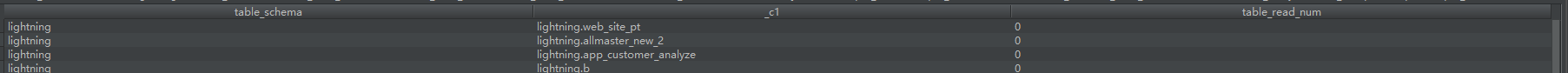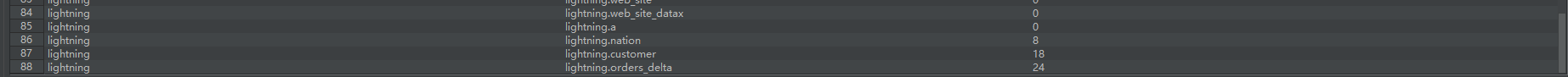https://h5.dingtalk.com/invite-page/index.html?bizSource=____source____&corpId=dingb682fb31ec15e09f35c2f4657eb6378f&inviterUid=E3F28CD2308408A8&encodeDeptId=0054DC2B53AFE745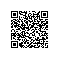使用钉钉扫一扫加入圈子
+ 订阅